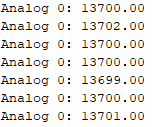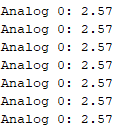Arduino ADC (Analogical - Digital Converter) board for industrial Arduino
ADS1115 16 bits ADC precision analog to digital converter with 16-bits of resolution
7 June, 2019 bySerzh Ohanyan### Introduction of this Arduino ADC

In this post, we talk about the Analogic-Digital Converter (ADC) 16-bits module ADS1115 for Arduino industrial automation controller. You will find some information about the module and the way to connect with industrial Arduino automation and read analog values.

## Requirements

### Parameter

 Power-Supply Voltage Storage Temperature Specified Temperature Analog Input Voltage Resolution Data Rate (DR) Data Rate Variation Supply Current Power dissipation

### Value

 2.0 to 5.5 V -60 to +150 ºC -40 to +125 ºC GND to VCC V 16 Bits 8, 16, 32, 64, 128, 250, 475, 860 SPS -10 to 10 % 150 - 200 uA VDD = 5.0, 3.3, 2.0 V

### Conditions

 - - - - - - All data rates Operating current at 25 ºC 0.9, 0.5, 0.3 mW

## Connections

According to Hardware Configuration (page 11) of the ADS115 Datasheet. The connections are the following:

## Code

The same code for Arduino Mega or M-Duino programmable logic controller Arduino

### ```#include <Wire.h> #include <Adafruit_ADS1015.h> Adafruit_ADS1115 ads; const float multiplier = 0.0001875F; void setup() { Serial.begin(9600);  // There are different GAINs // ads.setGain(GAIN_TWOTHIRDS); +/- 6.144V 1 bit = 0.1875mV (default) // ads.setGain(GAIN_ONE); +/- 4.096V 1 bit = 0.125mV // ads.setGain(GAIN_TWO); +/- 2.048V 1 bit = 0.0625mV // ads.setGain(GAIN_FOUR); +/- 1.024V 1 bit = 0.03125mV // ads.setGain(GAIN_EIGHT); +/- 0.512V 1 bit = 0.015625mV // ads.setGain(GAIN_SIXTEEN); +/- 0.256V 1 bit = 0.0078125mV ads.setGain(GAIN_TWOTHIRDS); ads.begin(); } void loop() { int16_t adc0; adc0 = ads.readADC_SingleEnded(0); Serial.print("Analog 0: ");   // Read value from 0 to 32767 from 16 bits register, so it's necessary to convert to real value // by multiplying by 0.0001875 (GAIN) Serial.println(adc0 * multiplier); delay(1000); }```

To test the program we connected the Power Supply to A0 at 2.5 V. That's the result in the Serial Monitor:

• With multiplier = 1. Decimal value of the 16 bit register.• With Multiplier = 0.0001875F. Decimal value of the 16 bit register multiplied by the appropriate multiplier to get the real value representation in V units.It is also possible to use the module ADS1115 to Raspberry Pi.Serzh Ohanyan
7 June, 2019